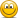P2.T8.20.2. Liquidity-adjusted value at risk (LVaR) (Hull Ch.24)

Nicole Seaman

Director of FRM Operations
Staff member
Subscriber
Learning objective: Explain and calculate liquidity trading risk via cost of liquidation and liquidity-adjusted VaR (LVaR).

Questions:

20.2.1. A portfolio holds 100,000 shares of a stock and this single position has a value of $3.0 million. The stock is quoted bid$29.00, offer $31.00. The stock's daily volatility is 1.43% or 143 basis points. For purposes of value at risk (VaR), we will assume the stock's arithmetic returns are normally distributed (aka, normal VaR) and the expected daily return rounds to zero (under these assumptions absolute VaR is identical to relative VaR). Which is NEAREST to the position's one-day 99.0% confident liquidity-adjusted value at risk (LVaR)? a.$15,000
b. $80,000 c.$200,000
d. $1.0 million 20.2.2. A position with a value of$2.0 million consists of 50,000 shares of stock. The stock is quoted bid $39.00, offer$41.00. The bid-offer spread has a volatility of 2.0%. The stock's daily volatility is 2.00% or 200 basis points. For purposes of value at risk (VaR), assume the stock's arithmetic returns are normally distributed (aka, normal VaR) and the expected daily return rounds to zero (under these assumptions absolute VaR is identical to relative VaR). We want to measure the cost of liquidity under stressed market conditions; aka, we will assume a worst-case volatile spread rather than a static spread. Which is NEAREST to the position's one-day 95.0% confident liquidity-adjusted value at risk (LVaR)? (Please assume a rounded normal deviate of 1.65).

a. $76,300 b.$149,000
c. $318,000 d.$755,000

20.2.3. A position with a value of $1.0 million consists of 50,000 shares of an asset. The asset is quoted bid$19.00, offer $21.00. The asset has an expected return of 14.0% per annual and an annual volatility of 26.0%. We are interested in the lognormal value at risk (aka, lognormal VaR) over a one-year horizon; that is, we assume geometric returns are normally distributed. If our confidence level is 95.0%, which is NEAREST to the one-year liquidity-adjusted lognormal VaR (LVaR)? a.$100,000
b. $200,000 c.$300,000
d. \$400,000

SunnyAmazon

New Member
But why I am not getting the answers for the LVar in question one

3m × 2.33× 0.0143 = 99957
Liquidity cost= 3m x 2/30 × 0.5 = 100000
LVaR = 100000 + 99957.

Please, see my logic and clarify

David Harper CFA FRM

David Harper CFA FRM
Staff member
Subscriber
@SunnyAmazon your logic is good!Please notice the question asks for the nearest ... let's see, your answer appears to be
almost exactly accurate while the correct choice is rounded and only, um, 0.021% away. Pretty close, I'd say!

SunnyAmazon

New Member
Oh, I didn't see that part. Thanks David